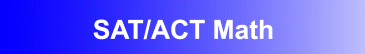Award-Winning

SAT ACT Math Prep and Score Improvement SoftwareDESCRIPTION
SAT ACT MATH is the ideal

software package for improving SAT ACT math scores.

Students learn problem solving skills and strategies that can greatly improve their performance on the tests.
SAT ACT MATH will help students master the particular types of problems presented in the Math sections of the SAT and ACT exams.
Designed by math educators and in accordance with math curriculum standards, the program helps students build problem solving skills and review the key concepts of arithmetic, algebra, and geometry.
Examples and problem solving exercises progress gradually from the simpler to the more challenging, allowing students to build their skills and confidence.
• Award-winning SAT and ACT preparation courseware developed by math educators
• Provides practice problems that are ideal for self-study, and as a supplement to classroom instruction
• Demonstrated success in boosting students' math skills and comprehension, and performance on the Math sections of the SAT and ACT exams
• Network versions contain student record-keeping, grading, and performance analysis features
AVAILABLE FOR WINDOWS
• Windows XP/Vista
• Windows 7/8
• Windows 10
PRICING Single User Version: \$49.95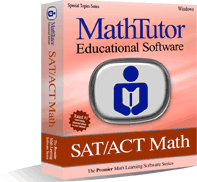TOPICS COVERED
Basic Math and Pre-Algebra
• Fractions: Comparisons
• Interpretation of Fractions
• Arithmetic Comparisons
• Proportions: Volume, Area, Length
Algebra
• Solving an Algebraic Equation I
• Solving an Algebraic Equation II
• Evaluating Algebraic Expressions
• Simplifying Algebraic Expressions
• Using Exponents
• Distance = Rate x Time
Geometry
Data Analysis, Functions, Other Topics
• Data Interpretation
• Representing Functions as Tables
• Functions: Comparisons
• Sequences
• Work Problems: Painting a House
• Averages: A Carpenter's Salary
• Averages of Test Scores
• Mixtures: Salt Water
• Absolute Value
• Word Problems: Factory Workers
• Word Problems: People at a Party
• Word Problems: Packages
• Probability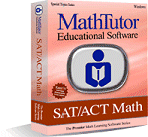Order Now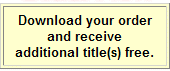TOPICS COVERED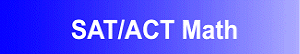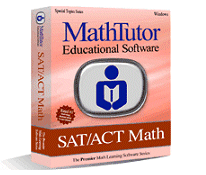TOPICS COVERED×